# Telemetry Decoder

The role of a Telemetry Decoder block is to obtain the data bits from the navigation message broadcast by GNSS satellites.

In the description of baseband signals, this page uses the following notation:

• $$[l]_{L}$$ means the integer part of $$\frac{l}{L}$$,
• $$\oplus$$ is the exclusive–or operation (modulo–2 addition), and
• $$\mid l \mid_{L}$$ means $$l$$ modulo $$L$$.

### Implementation: GPS_L1_CA_Telemetry_Decoder

The GPS L1 C/A baseband signal can be written as:

$\begin{equation} s^{\text{(GPS L1)}}_{T}(t) = e_{L1I}(t) + j e_{L1Q}(t)~, \end{equation}$

with

$\begin{eqnarray} e_{L1I}(t) & = & \sum_{l=-\infty}^{\infty} {\color{ForestGreen} D_{\text{NAV}}\Big[ [l]_{204600}\Big]} \oplus C_{\text{P(Y)}} \Big[ |l|_{L_{\text{P(Y)}}} \Big] \cdot p(t - lT_{c,\text{P(Y)}})~,\\ e_{L1Q}(t) & = & \sum_{l=-\infty}^{\infty} {\color{ForestGreen} D_{\text{NAV}}\Big[ [l]_{20460}}\Big] \oplus C_{\text{C/A}} \Big[ |l|_{1023} \Big] \cdot p(t - lT_{c,\text{C/A}})~. \end{eqnarray}$

The GPS NAV message $$D_{\text{NAV}} \in \{ 1, -1 \}$$ is modulated at 50 bits per second. The whole message contains 25 pages (or “frames”) of 30 seconds each, forming the master frame that takes 12,5 minutes to be transmitted. Every frame is subdivided into 5 sub-frames of 6 seconds each; in turn, every sub-frame consists of 10 words, with 30 bits per word: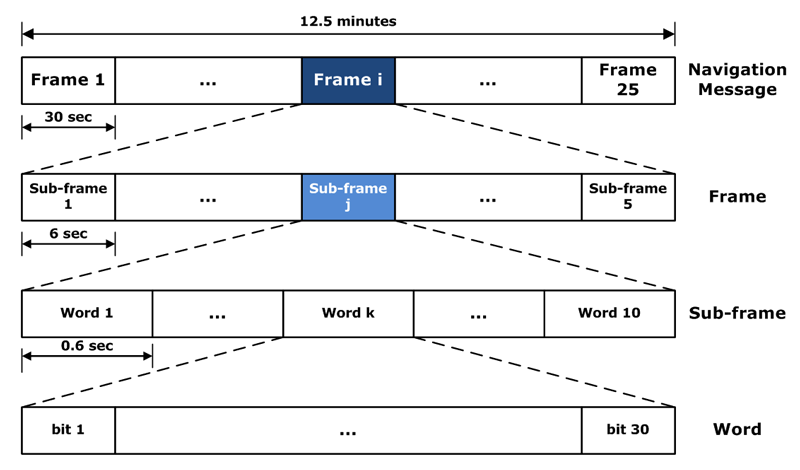GPS NAV message. Source: Navipedia.

The content of every sub-frame is as follows:

• Sub-frame 1: contains information about the parameters to be applied to satellite clock status for its correction. These values are polynomial coefficients that allow converting time onboard to GPS time. It also has information about the satellite’s health condition.
• Sub-frames 2 and 3: these sub-frames contain satellite ephemeris.
• Sub-frame 4: provides ionospheric model parameters (in order to adjust for ionospheric refraction), UTC information (Universal Coordinate Time), part of the almanac, and indications of whether the Anti-Spoofing, A/S, is activated or not (which transforms P code into the encrypted Y code).
• Sub-frame 5: contains data from the almanac and the constellation status. A total of 25 frames are needed to complete the almanac.

Sub-frames 1, 2, and 3 are transmitted with each frame (i.e., they are repeated every 30 seconds). Sub-frames 4 and 5 contain different pages (25 pages each) of the navigation message. Thence, the transmission of the full navigation message takes $$25 \times 30$$ seconds = 12.5 minutes.

The content of sub-frames 4 and 5 is common for all satellites. Thence, the almanac data for all in-orbit satellites can be obtained from a single tracked satellite.

This implementation accepts the following parameters:

Parameter Description Required
implementation GPS_L1_CA_Telemetry_Decoder Mandatory
dump [true, false]: If set to true, it enables the Telemetry Decoder internal binary data file logging (see section Binary Output down below for details). It defaults to false. Optional
dump_filename If dump is set to true, base name of the files in which internal data will be stored. It defaults to ./telemetry, so files will be named ./telemetryN, where N is the channel number (automatically added). Optional
dump_mat [true, false]: If dump is set to true, the binary output is converted to .mat format, readable from Matlab7octave and Python, at the end of the receiver execution. By default, it is set to the same value as dump. Optional
remove_dat [true, false]: If dump=true and dump_mat is not set, or set to true, then this parameter controls if the internal .dat binary file is removed after conversion to .mat, leaving a cleaner output if the user is not interested in the .dat file. By default, this parameter is set to false. Optional
dump_crc_stats [true, false]: If set to true, the success rate of the CRC check when decoding navigation messages is reported in a file generated at the end of the processing (or when exiting with q + [Enter]). By default, this parameter is set to false. Optional
dump_crc_stats_filename If dump_crc_stats=true, this parameter sets the base name of the files in which the CRC success rate is reported. It defaults to telemetry_crc_stats, so files named telemetry_crc_stats_chN.txt will be created, with N in chN being the channel number. Optional

Telemetry Decoder implementation: GPS_L1_CA_Telemetry_Decoder.

Example:

;######### TELEMETRY DECODER CONFIG FOR GPS L1 CHANNELS ############
TelemetryDecoder_1C.implementation=GPS_L1_CA_Telemetry_Decoder
TelemetryDecoder_1C.dump=false


### Implementation: Galileo_E1B_Telemetry_Decoder

The Galileo E1 baseband signal can be written as:

$\begin{eqnarray} s^{\text{(Gal E1)}}_{T}(t)& = &\frac{1}{\sqrt{2}} \Big(e_{E1B}(t)\left(\alpha sc_A(t) + \beta sc_B(t) \right) + \nonumber \\ {} & {} & -~e_{E1C}(t) \left(\alpha sc_A(t) - \beta sc_B(t) \right) \Big)~, \end{eqnarray}$

where $$sc_A(t)$$ and $$sc_B(t)$$ are the Composite Binary Offset Carrier (CBOC) square subcarriers, and:

$\begin{equation} e_{E1B}(t) = \sum_{l=-\infty}^{+\infty} {\color{ForestGreen} D_{\text{I/NAV}} \Big[ [l]_{4092}\Big]} \oplus C_{E1B}\Big[|l|_{4092}\Big] p(t - lT_{c,E1B})~. \end{equation}$

As shown in this equation, the E1B signal component carries the $$D_{\text{I/NAV}} \in \{ 1, -1 \}$$ navigation message, which provides the space vehicle identification (SVID), an Issue of Data, the ephemeris data, a signal-in-space accuracy (SISA) indicator, clock correction parameters, an ionospheric correction, the Broadcast Group Delay (BGD), signal health and data validity status, Galileo System Time (GST), GST-UTC and GST-GPS time conversion parameters, the almanacs, and Search and Rescue (SAR) service data.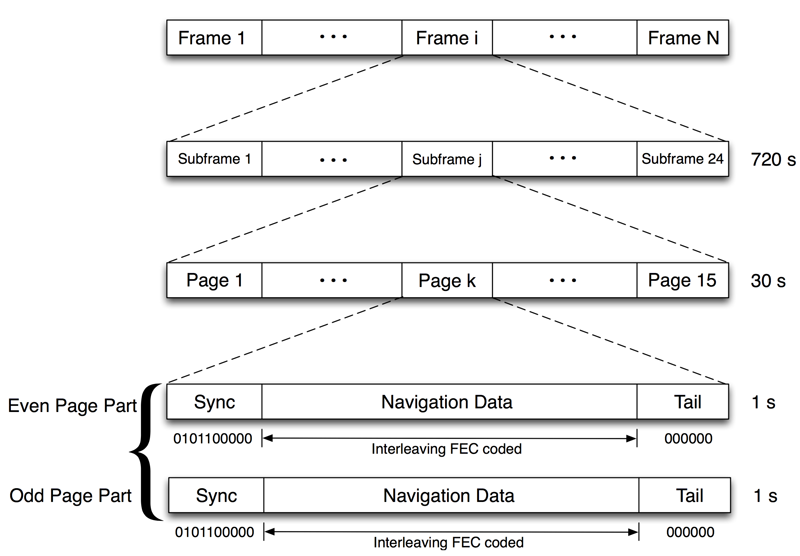Galileo E1B I/NAV message structure.

In the message structure depicted above, each frame contains 24 subframes, and each subframe contains 15 nominal pages, having a duration of 2 seconds transmitted sequentially in time in two parts of duration 1 second each. Each page part (denoted as even or odd) consists of a 10-bit synchronization pattern followed by 240 coded symbols, corresponding to 114 data bits and 6 tail bits (sequence of zeros) that allow Viterbi decoding. Three levels of error coding are applied to the Galileo message data stream:

• a Cyclic Redundancy Check (CRC) with error detection capabilities after recovery of the received data,

• a one-half rate Forward Error Correction (FEC) convolutional coding, and

• block interleaving on the resulting frames, with $$30$$ columns (where data is written) and $$8$$ rows (where data is read), providing robustness to the FEC decoding algorithm by avoiding error bursts.

This implementation accepts the following parameters:

Parameter Description Required
implementation Galileo_E1B_Telemetry_Decoder Mandatory
enable_reed_solomon [true, false]: If set to true, it enables the FEC2 Erasure Correction defined for the Galileo E1B INAV message at OS ICD v2.0. It defaults to false. Optional
use_reduced_ced [true, false]: If set to true, it enables the use of the reduced CED parameters transmitted in Galileo E1B INAV message. It defaults to false. This configuration parameter is only present on the next branch of the upstream repository, and it will be included in the next stable release of GNSS-SDR. Optional
dump [true, false]: If set to true, it enables the Telemetry Decoder internal binary data file logging (see section Binary Output down below for details). It defaults to false. Optional
dump_filename If dump is set to true, base name of the files in which internal data will be stored. It defaults to ./telemetry, so files will be named ./telemetryN, where N is the channel number (automatically added). Optional
dump_mat [true, false]: If dump is set to true, the binary output is converted to .mat format, readable from Matlab7octave and Python, at the end of the receiver execution. By default, it is set to the same value as dump. Optional
remove_dat [true, false]: If dump=true and dump_mat is not set, or set to true, then this parameter controls if the internal .dat binary file is removed after conversion to .mat, leaving a cleaner output if the user is not interested in the .dat file. By default, this parameter is set to false. Optional
dump_crc_stats [true, false]: If set to true, the success rate of the CRC check when decoding navigation messages is reported in a file generated at the end of the processing (or when exiting with q + [Enter]). By default, this parameter is set to false. Optional
dump_crc_stats_filename If dump_crc_stats=true, this parameter sets the base name of the files in which the CRC success rate is reported. It defaults to telemetry_crc_stats, so files named telemetry_crc_stats_chN.txt will be created, with N in chN being the channel number. Optional

Telemetry Decoder implementation: Galileo_E1B_Telemetry_Decoder.

Example:

;######### TELEMETRY DECODER CONFIG FOR GALILEO E1 CHANNELS ############
TelemetryDecoder_1B.implementation=Galileo_E1B_Telemetry_Decoder
TelemetryDecoder_1B.dump=false


### Implementation: Galileo_E5b_Telemetry_Decoder

The Galileo E5b signal can be expressed as:

$\begin{equation} s_{T}^{(Gal E5b)}(t) \simeq e_{E5bI}(t)+je_{E5bQ}(t). \end{equation}$

where:

$\begin{eqnarray} e_{E5bI}(t) & = & \sum_{m=-\infty}^{+\infty}C_{E5bIs}\Big[|m|_{4}\Big] \oplus \sum_{l=1}^{10230}C_{E5aIp}\Big[ l \Big] \oplus \nonumber \\ {} & {} & \oplus~ {\color{ForestGreen} D_{\text{I/NAV}} \Big[ [l]_{40920}\Big]} p(t-mT_{c,E5s}-lT_{c,E5p})~, \end{eqnarray}$ $\begin{eqnarray} e_{E5bQ}(t) & = & \sum_{m=-\infty}^{+\infty}C_{E5bQs}\Big[|m|_{100}\Big] \oplus \sum_{l=1}^{10230}C_{E5bQp}\Big[ l \Big] \cdot\nonumber \\ {} & {} & \cdot~p(t-mT_{c,E5s}-lT_{c,E5p})~, \end{eqnarray}$

As shown in these equations, the E5b signal carries the $$D_{\text{I/NAV}} \in \{1, -1 \}$$ navigation message in its Inphase component.

This implementation accepts the following parameters:

Parameter Description Required
implementation Galileo_E5b_Telemetry_Decoder Mandatory
dump [true, false]: If set to true, it enables the Telemetry Decoder internal binary data file logging (see section Binary Output down below for details). It defaults to false. Optional
dump_filename If dump is set to true, base name of the files in which internal data will be stored. It defaults to ./telemetry, so files will be named ./telemetryN, where N is the channel number (automatically added). Optional
dump_mat [true, false]: If dump is set to true, the binary output is converted to .mat format, readable from Matlab7octave and Python, at the end of the receiver execution. By default, it is set to the same value as dump. Optional
remove_dat [true, false]: If dump=true and dump_mat is not set, or set to true, then this parameter controls if the internal .dat binary file is removed after conversion to .mat, leaving a cleaner output if the user is not interested in the .dat file. By default, this parameter is set to false. Optional
dump_crc_stats [true, false]: If set to true, the success rate of the CRC check when decoding navigation messages is reported in a file generated at the end of the processing (or when exiting with q + [Enter]). By default, this parameter is set to false. Optional
dump_crc_stats_filename If dump_crc_stats=true, this parameter sets the base name of the files in which the CRC success rate is reported. It defaults to telemetry_crc_stats, so files named telemetry_crc_stats_chN.txt will be created, with N in chN being the channel number. Optional

Telemetry Decoder implementation: Galileo_E5b_Telemetry_Decoder.

Example:

TelemetryDecoder_7X.implementation=Galileo_E5b_Telemetry_Decoder
TelemetryDecoder_7X.dump=false


The complex baseband transmitted signal by GLONASS satellites in the L1 and L2 bands can be written as

$\begin{equation} s^{\text{(GLO)}}_{T}(t) = e_{I}(t) + j e_{Q}(t)~, \end{equation}$

where

$\begin{equation} e_{Q}(t) = \sum_{l=-\infty}^{\infty} {\color{ForestGreen} D_{\text{GNAV}}\Big[ [l]_{10220} \Big] } \oplus C_{\text{C/A}} \Big[ |l|_{511} \Big] p(t - lT_{c,\text{C/A}})~. \end{equation}$

The navigation message of the standard accuracy signal (C/A) is broadcast as continuously repeating superframes with a duration of 2.5 minutes. Each superframe consists of 5 frames of 30 seconds, and each frame consists of 15 strings of 2 seconds duration (100 bits long).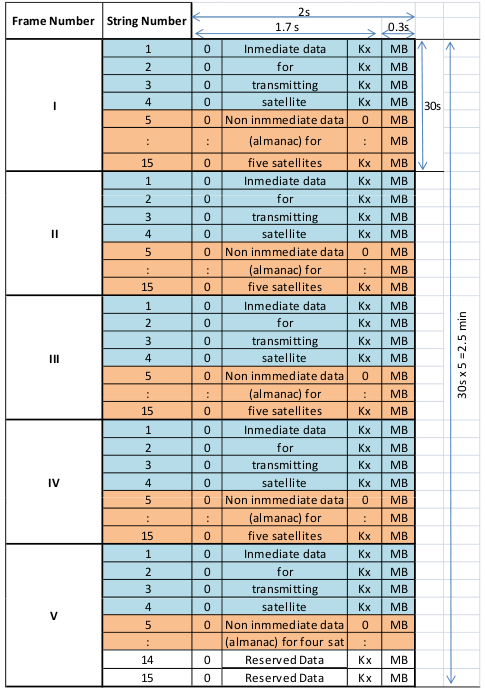GLONASS NAV message. Source: Navipedia.

Each string is formed by a 0 (idle) bit, 76 data bits, the eight check bits of a Hamming code (labeled as Kx in the figure above), and a 30-bit time mark (labeled as MB).

The message content divides the data into immediate data of the transmitting satellite and non-immediate data for the other satellites:

• The immediate data is repeated in the first four strings of every frame. It comprises the ephemeris parameters, satellite clock offsets, satellite healthy flag, and the relative difference between the carrier frequency of the satellite and its nominal value.
• The non-immediate data is broadcast in the strings 5 to 15 of each frame (almanac for 24 satellites). The frames I to IV contain almanac for 20 satellites (5 per frame), and the 5th frame almanac for 4 satellites. The last 2 strings of frame 5 are reserved bits (the almanac of each satellite uses 2 strings).

The ephemerides values are predicted from the Ground Control Centre for a 24 hours period, and the satellite transmits a new set of ephemerides every 30 minutes. These data differ from GPS data: instead of Keplerian orbital elements, they are provided as Earth Centered Earth Fixed (ECEF) Cartesian coordinates in position and velocity, with lunar and solar acceleration perturbation parameters.

### Implementation: GLONASS_L1_CA_Telemetry_Decoder

This implementation accepts the following parameters:

Parameter Description Required
implementation GLONASS_L1_CA_Telemetry_Decoder Mandatory
dump [true, false]: If set to true, it enables the Telemetry Decoder internal binary data file logging (see section Binary Output down below for details). It defaults to false. Optional
dump_filename If dump is set to true, base name of the files in which internal data will be stored. It defaults to ./telemetry, so files will be named ./telemetryN, where N is the channel number (automatically added). Optional
dump_mat [true, false]: If dump is set to true, the binary output is converted to .mat format, readable from Matlab7octave and Python, at the end of the receiver execution. By default, it is set to the same value as dump. Optional
remove_dat [true, false]: If dump=true and dump_mat is not set, or set to true, then this parameter controls if the internal .dat binary file is removed after conversion to .mat, leaving a cleaner output if the user is not interested in the .dat file. By default, this parameter is set to false. Optional
dump_crc_stats [true, false]: If set to true, the success rate of the CRC check when decoding navigation messages is reported in a file generated at the end of the processing (or when exiting with q + [Enter]). By default, this parameter is set to false. Optional
dump_crc_stats_filename If dump_crc_stats=true, this parameter sets the base name of the files in which the CRC success rate is reported. It defaults to telemetry_crc_stats, so files named telemetry_crc_stats_chN.txt will be created, with N in chN being the channel number. Optional

Telemetry Decoder implementation: GLONASS_L1_CA_Telemetry_Decoder.

Example:

;######### TELEMETRY DECODER CONFIG FOR GLONASS L1 CHANNELS ############
TelemetryDecoder_1G.implementation=GLONASS_L1_CA_Telemetry_Decoder
TelemetryDecoder_1G.dump=false


### Implementation: GLONASS_L2_CA_Telemetry_Decoder

This implementation accepts the following parameters:

Parameter Description Required
implementation GLONASS_L2_CA_Telemetry_Decoder Mandatory
dump [true, false]: If set to true, it enables the Telemetry Decoder internal binary data file logging (see section Binary Output down below for details). It defaults to false. Optional
dump_filename If dump is set to true, base name of the files in which internal data will be stored. It defaults to ./telemetry, so files will be named ./telemetryN, where N is the channel number (automatically added). Optional
dump_mat [true, false]: If dump is set to true, the binary output is converted to .mat format, readable from Matlab7octave and Python, at the end of the receiver execution. By default, it is set to the same value as dump. Optional
remove_dat [true, false]: If dump=true and dump_mat is not set, or set to true, then this parameter controls if the internal .dat binary file is removed after conversion to .mat, leaving a cleaner output if the user is not interested in the .dat file. By default, this parameter is set to false. Optional
dump_crc_stats [true, false]: If set to true, the success rate of the CRC check when decoding navigation messages is reported in a file generated at the end of the processing (or when exiting with q + [Enter]). By default, this parameter is set to false. Optional
dump_crc_stats_filename If dump_crc_stats=true, this parameter sets the base name of the files in which the CRC success rate is reported. It defaults to telemetry_crc_stats, so files named telemetry_crc_stats_chN.txt will be created, with N in chN being the channel number. Optional

Telemetry Decoder implementation: GLONASS_L2_CA_Telemetry_Decoder.

Example:

;######### TELEMETRY DECODER CONFIG FOR GLONASS L2 CHANNELS ############
TelemetryDecoder_2G.implementation=GLONASS_L2_CA_Telemetry_Decoder
TelemetryDecoder_2G.dump=true


### Implementation: GPS_L2C_Telemetry_Decoder

The GPS L2C baseband signal can be written as:

$\begin{equation} s^{\text{(GPS L2)}}_{T}(t) = e_{L2I}(t) + j e_{L2Q}(t)~, \end{equation}$

with the Quadrature–phase component defined as:

$\begin{eqnarray} e_{L2Q}(t) & = & \sum_{l=-\infty}^{\infty} {\color{ForestGreen} D_{\text{CNAV}} \Big[ [l]_{10230} \Big] } \oplus \left(C_{\text{CL}} \Big[ |l|_{L_{\text{CL}}} \Big] p_{\text{1/2}} \left(t - lT_{c,L2C} \right) + \right. \nonumber \\ {} & {} & + \left. C_{\text{CM}} \Big[ |l|_{L_{\text{CM}}} \Big] p_{\text{1/2}}\left(t - \left(l + \frac{3}{4}\right)T_{c,L2C}\right) \right)~. \end{eqnarray}$

The civilian long code $$C_{\text{CL}}$$ is $$L_{\text{CL}}=767250$$ chips long, repeating every $$1.5$$ s, while the civilian moderate code $$C_{\text{CM}}$$ is $$L_{\text{CL}}=10230$$ chips long and it repeats every $$20$$ ms. The CNAV data message $$D_{\text{CNAV}} \in \{ 1, -1 \}$$ is an upgraded version of the original NAV navigation message, containing higher precision representation and nominally more accurate data than the NAV data. It is transmitted at $$25$$ bps with forward error correction (FEC) encoding, resulting in $$50$$ sps.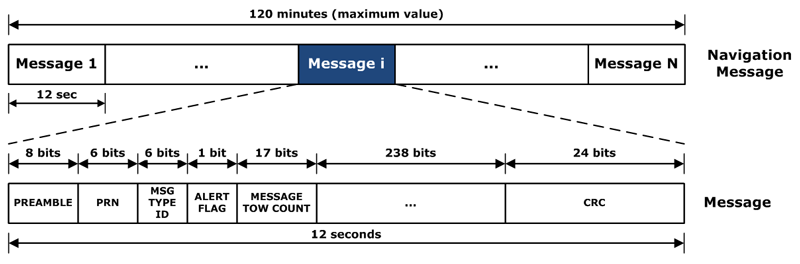GPS L2 CNAV message structure. Source: Navipedia.

This implementation accepts the following parameters:

Parameter Description Required
implementation GPS_L2C_Telemetry_Decoder Mandatory
dump [true, false]: If set to true, it enables the Telemetry Decoder internal binary data file logging (see section Binary Output down below for details). It defaults to false. Optional
dump_filename If dump is set to true, base name of the files in which internal data will be stored. It defaults to ./telemetry, so files will be named ./telemetryN, where N is the channel number (automatically added). Optional
dump_mat [true, false]: If dump is set to true, the binary output is converted to .mat format, readable from Matlab7octave and Python, at the end of the receiver execution. By default, it is set to the same value as dump. Optional
remove_dat [true, false]: If dump=true and dump_mat is not set, or set to true, then this parameter controls if the internal .dat binary file is removed after conversion to .mat, leaving a cleaner output if the user is not interested in the .dat file. By default, this parameter is set to false. Optional
dump_crc_stats [true, false]: If set to true, the success rate of the CRC check when decoding navigation messages is reported in a file generated at the end of the processing (or when exiting with q + [Enter]). By default, this parameter is set to false. Optional
dump_crc_stats_filename If dump_crc_stats=true, this parameter sets the base name of the files in which the CRC success rate is reported. It defaults to telemetry_crc_stats, so files named telemetry_crc_stats_chN.txt will be created, with N in chN being the channel number. Optional

Telemetry Decoder implementation: GPS_L2C_Telemetry_Decoder.

Example:

;######### TELEMETRY DECODER CONFIG FOR GPS L2C CHANNELS ############
TelemetryDecoder_2S.implementation=GPS_L2C_Telemetry_Decoder
TelemetryDecoder_2S.dump=true


### Implementation: GPS_L5_Telemetry_Decoder

The GPS L5 baseband signal can be written as:

$\begin{equation} s^{\text{(GPS L5)}}_{T}(t) = e_{L5I}(t) + j e_{L5Q}(t)~, \end{equation}$

where:

$\begin{eqnarray} e_{L5I}(t) & = & \sum_{m=-\infty}^{+\infty} C_{nh_{10}} \Big[ |m|_{10}\Big] \oplus {\color{ForestGreen} D_{\text{CNAV}}\Big[ [m]_{10}\Big]} \oplus \nonumber \\ {} & {} & \oplus~\sum_{l=1}^{102300} C_{L5I}\Big[|l|_{10230}\Big] \cdot p(t - m T_{c,nh} - lT_{c,L5}) ~, \end{eqnarray}$ $\!\!\!\!\!\!\!\!\begin{equation} e_{L5Q}(t) \! = \!\! \sum_{m=-\infty}^{+\infty}\!\! C_{nh_{20}} \Big[ |m|_{20}\Big] \! \oplus \!\! \sum_{l=1}^{102300}\!\!C_{L5Q}\Big[|l|_{10230}\Big] \cdot p(t \! - \! m T_{c,nh} \! - \! lT_{c,L5})~, \end{equation}$

with $$T_{c,nh}=1$$ ms and $$T_{c,L5}=\frac{1}{10.23}$$ $$\mu$$s. The L5I component contains a synchronization sequence $$C_{nh_{10}}$$ that modulates each $$100$$ symbols of the GPS L5 civil navigation data $$D_{\text{CNAV}}$$. The message structure is the same as for L2 CNAV: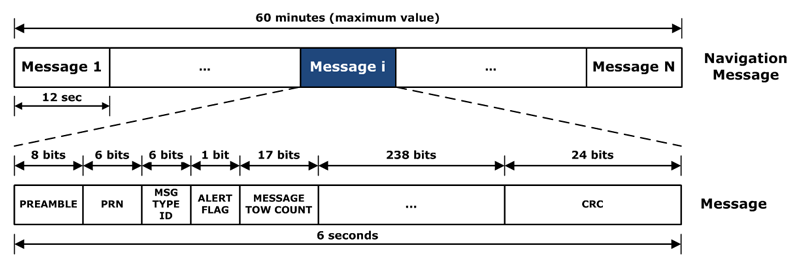GPS L5 CNAV message structure. Source: Navipedia.

This implementation accepts the following parameters:

Parameter Description Required
implementation GPS_L5_Telemetry_Decoder Mandatory
dump [true, false]: If set to true, it enables the Telemetry Decoder internal binary data file logging (see section Binary Output down below for details). It defaults to false. Optional
dump_filename If dump is set to true, base name of the files in which internal data will be stored. It defaults to ./telemetry, so files will be named ./telemetryN, where N is the channel number (automatically added). Optional
dump_mat [true, false]: If dump is set to true, the binary output is converted to .mat format, readable from Matlab7octave and Python, at the end of the receiver execution. By default, it is set to the same value as dump. Optional
remove_dat [true, false]: If dump=true and dump_mat is not set, or set to true, then this parameter controls if the internal .dat binary file is removed after conversion to .mat, leaving a cleaner output if the user is not interested in the .dat file. By default, this parameter is set to false. Optional
dump_crc_stats [true, false]: If set to true, the success rate of the CRC check when decoding navigation messages is reported in a file generated at the end of the processing (or when exiting with q + [Enter]). By default, this parameter is set to false. Optional
dump_crc_stats_filename If dump_crc_stats=true, this parameter sets the base name of the files in which the CRC success rate is reported. It defaults to telemetry_crc_stats, so files named telemetry_crc_stats_chN.txt will be created, with N in chN being the channel number. Optional

Telemetry Decoder implementation: GPS_L5_Telemetry_Decoder.

Example:

;######### TELEMETRY DECODER CONFIG FOR GPS L5 CHANNELS ############
TelemetryDecoder_L5.implementation=GPS_L5_Telemetry_Decoder
TelemetryDecoder_L5.dump=true


### Implementation: Galileo_E5a_Telemetry_Decoder

The Galileo E5 baseband signal can be written as:

$\begin{eqnarray} s^{\text{(Gal E5)}}_{T}(t) & = & e_{E5a}(t) ssc_s^{*}(t) + e_{E5b}(t) ssc_s(t) + \nonumber \\ {} & {} & +~\bar{e}_{E5a}(t)ssc_p^{*}(t)+\bar{e}_{E5b}(t)ssc_p(t)~, \end{eqnarray}$

where $$ssc_s(t)$$ and $$ssc_p(t)$$ are the single and product side–band signal subcarriers. However, sub-band E5a can be approximated by a QPSK signal. Galileo’s F/NAV navigation message $$D_{\text{F/NAV}} \in \{ 1, -1 \}$$ modulates the I component of the E5a signal, which can be expressed as:

$\begin{eqnarray} e_{E5aI}(t) & = & \sum_{m=-\infty}^{+\infty}C_{E5aIs}\Big[|m|_{20}\Big] \oplus \sum_{l=1}^{10230}C_{E5aIp}\Big[ l \Big] \oplus \nonumber \\ {} & {} & \oplus~{\color{ForestGreen} D_{\text{F/NAV}} \Big[ [l]_{204600}\Big]} \cdot p(t - mT_{c,E5s} - lT_{c,E5p})~. \end{eqnarray}$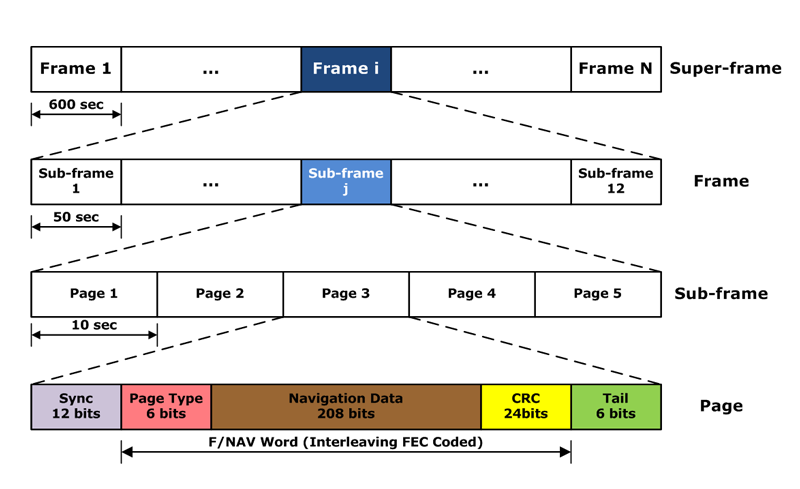Galileo E5a F/NAV message structure. Source: Navipedia.

This implementation accepts the following parameters:

Parameter Description Required
implementation Galileo_E5a_Telemetry_Decoder Mandatory
dump [true, false]: If set to true, it enables the Telemetry Decoder internal binary data file logging (see section Binary Output down below for details). It defaults to false. Optional
dump_filename If dump is set to true, base name of the files in which internal data will be stored. It defaults to ./telemetry, so files will be named ./telemetryN, where N is the channel number (automatically added). Optional
dump_mat [true, false]: If dump is set to true, the binary output is converted to .mat format, readable from Matlab7octave and Python, at the end of the receiver execution. By default, it is set to the same value as dump. Optional
remove_dat [true, false]: If dump=true and dump_mat is not set, or set to true, then this parameter controls if the internal .dat binary file is removed after conversion to .mat, leaving a cleaner output if the user is not interested in the .dat file. By default, this parameter is set to false. Optional
dump_crc_stats [true, false]: If set to true, the success rate of the CRC check when decoding navigation messages is reported in a file generated at the end of the processing (or when exiting with q + [Enter]). By default, this parameter is set to false. Optional
dump_crc_stats_filename If dump_crc_stats=true, this parameter sets the base name of the files in which the CRC success rate is reported. It defaults to telemetry_crc_stats, so files named telemetry_crc_stats_chN.txt will be created, with N in chN being the channel number. Optional

Telemetry Decoder implementation: Galileo_E5a_Telemetry_Decoder.

Example:

;######### TELEMETRY DECODER CONFIG FOR GALILEO E5a CHANNELS ############
TelemetryDecoder_5X.implementation=Galileo_E5a_Telemetry_Decoder
TelemetryDecoder_5X.dump=false


### Implementation: Galileo_E6_Telemetry_Decoder

NOTE: This block implementation is only available from the next branch of the upstream repository. It will be available in the next stable release.

The Galileo $$e_{E6B}(t)$$ baseband signal component can be written as:

$\begin{equation} e_{E6B}(t) = \sum_{m=-\infty}^{+\infty} {\color{ForestGreen}D_{\text{HAS}} \Big[ [m]_{5115}\Big]} \oplus C_{E6B}\Big[|m|_{5115}\Big] \cdot p(t - mT_{c,E6B})~, \end{equation}$

where $$D_{\text{HAS}}$$ is the High Accuracy Service data stream, which is modulated with the ranging code $$C_{E6B}$$ with chip period $$T_{c,E6B}=\frac{1}{5.115}$$ $$\mu$$s.

This implementation accepts the following parameters:

Parameter Description Required
implementation Galileo_E6_Telemetry_Decoder Mandatory
dump [true, false]: If set to true, it enables the Telemetry Decoder internal binary data file logging (see section Binary Output down below for details). It defaults to false. Optional
dump_filename If dump is set to true, base name of the files in which internal data will be stored. It defaults to ./telemetry, so files will be named ./telemetryN, where N is the channel number (automatically added). Optional
dump_mat [true, false]: If dump is set to true, the binary output is converted to .mat format, readable from Matlab7octave and Python, at the end of the receiver execution. By default, it is set to the same value as dump. Optional
remove_dat [true, false]: If dump=true and dump_mat is not set, or set to true, then this parameter controls if the internal .dat binary file is removed after conversion to .mat, leaving a cleaner output if the user is not interested in the .dat file. By default, this parameter is set to false. Optional
dump_crc_stats [true, false]: If set to true, the success rate of the CRC check when decoding navigation messages is reported in a file generated at the end of the processing (or when exiting with q + [Enter]). By default, this parameter is set to false. Optional
dump_crc_stats_filename If dump_crc_stats=true, this parameter sets the base name of the files in which the CRC success rate is reported. It defaults to telemetry_crc_stats, so files named telemetry_crc_stats_chN.txt will be created, with N in chN being the channel number. Optional

Telemetry Decoder implementation: Galileo_E6_Telemetry_Decoder.

Example:

;######### TELEMETRY DECODER CONFIG FOR GALILEO E6B CHANNELS ############
TelemetryDecoder_E6.implementation=Galileo_E6_Telemetry_Decoder
TelemetryDecoder_E6.dump=false
TelemetryDecoder_E6.dump_filename=has_data
TelemetryDecoder_E6.remove_dat=true
TelemetryDecoder_E6.dump_crc_stats=false
TelemetryDecoder_E6.dump_crc_stats_filename=./e6_stats/crc_stats


## Binary output

In all Telemetry Decoder blocks, if dump=true, the logging of internal processing data is also delivered in MATLAB Level 5 MAT-file v7.3 format, in a file with the same name than dump_filename but terminated in .mat instead of .dat. This is a compressed binary file format that can be easily read with Matlab or Octave, by doing load telemetryN.mat, where N is the channel number, or in Python via the h5py library. The stored variables are vectors with a number of columns equal to the total number of epochs (that is, tracking integration times) processed by the Telemetry Decoder block. The blocks store the following variables:

• TOW_at_current_symbol_ms: Time of Week associated with the current symbol for each epoch, in ms (different granularity depending on the message structure for each particular signal). Data type: double.
• tracking_sample_counter: Sample counter associated with each epoch. Data type: uint64_t.
• TOW_at_Preamble_ms: Time of Week associated with the preamble of the current symbol for each epoch, in ms (different granularity depending on the message structure for each particular signal). Data type: double.
• nav_symbol: Navigation message symbol $$\{ \pm 1 \}$$, as obtained by the Tracking block, for each epoch. Data type: int32_t.
• PRN: Satellite ID processed in each epoch. Data type: int32_t.

Examples:

1.- Retrieve the .dat and.mat files with nav_data base name (with XX being 1C, 1B, 1G, 2G, 2S, L5, or 5X):

TelemetryDecoder_XX.dump=true
TelemetryDecoder_XX.dump_filename=nav_data


so files will be named nav_data0.dat, nav_data0.mat, nav_data1.dat, nav_data1.mat, etc.

2.- Retrieve the .mat files only:

TelemetryDecoder_XX.dump=true
TelemetryDecoder_XX.remove_dat=true


so files will be named telemetry0.mat, telemetry1.mat, etc.

3.- Retrieve the .dat files only:

TelemetryDecoder_XX.dump=true
TelemetryDecoder_XX.dump_filename=nav_data
TelemetryDecoder_XX.dump_mat=false


so files will be named nav_data0.dat, nav_data1.dat, etc.

Retrieving the decoded bits of the navigation message (that is, the navigation data bits after all the required decoding mechanisms, including deinterleaving, FEC decoding, etc.), so they appear as described in their corresponding ICDs, is an interesting feature for educational and research purposes. To this end, there is a Navigation Data monitor that is able to forward decoded navigation messages to any IP addresses via UDP. In order to enable this option, all you need to do is including these three lines in your configuration file:

NavDataMonitor.enable_monitor=true
NavDataMonitor.port=1237                   ; destination port


where 127.0.0.1 is your desired destination IP and 1237 is your desired destination IP port (those are the default values). You can specify multiple destination addresses, separated by underscores:

NavDataMonitor.client_addresses=79.154.253.31_79.154.253.32


This applies to all existing Telemetry Decoder implementations.

Then, you need a listener application running at the destination address. All you need for developing your own listener application is the nav_message.proto file, which you are free to copy and use according to its 3-Clause BSD license, the Protocol Buffers library, also with a similar license, and a library to handle UDP/IP communications.

The Protocol Buffers library allows to write listener applications in a wide range of programming languages. A very simple example written in C++ (an application that listens to a given port and dumps the retrieved messages in the terminal) using the nav_message.proto file is provided here. This example uses the Boost libraries to handle UDP communications with Boost Asio.

Updated: